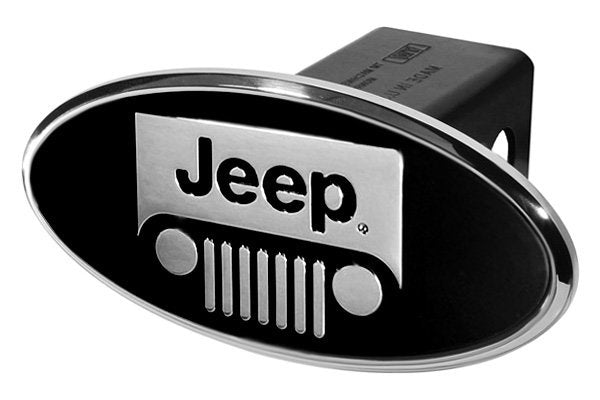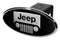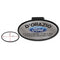Hitch Covers

• Made of rugged, durable plastic that snaps into place
• Printing is domed to give high quality 3-D effect and covered with urethane coating
• Available for 1" and 2" hitches

Pricing:

• 100= 4.95 each
• 250= 3.65 each
• 500= 3.20 each
• 1000= 2.90 each

• 100= .30 each
• 250= .27 each
• 500= .21 each
• 1000= .16 each

4 color process:

• 100= 5.65 each
• 250= 4.65 each
• 500= 3.80 each
• 1000= 3.30 each

*\$45 setup charge

*3-4 week production time

*All orders subject to +/- 5% overrun